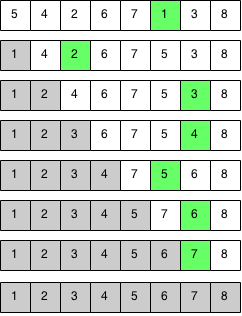## Introduction

Selection sort works by finding the smallest element in the array and swapping it with the first element. Then it finds the second smallest element and swaps it with the second element and it does this until the array is sorted.

## Implementation

We keep picking the smallest element and swapping it with the current position until the array is sorted.### Code

```public static void selectionSort(int array[]) {
// Iterates through the array selecting the smallest elements
for (int i = 0; i < array.length - 1; i++) {
int minIndex = i;

for (int j = i + 1; j < array.length; j++) {
// Find the index of the smallest element from i to n.
if (array[j] < array[minIndex]) {
minIndex = j;
}
// Swap the smallest element with the element at i.
int temp = array[i];
array[i] = array[minIndex];
array[minIndex] = temp;
}
}
}

```